# C++ Program to Print Star and Pyramid Patterns

In this article, you will learn and get code to print many types of patterns using stars (*), numbers, and alphabets to create pyramid, triangle, etc.-like shapes. Here is the list of pattern programs in C++:

And a lot more programs are available in this article. Let's start with the very first one, that is, the printing of a half-pyramid pattern using stars (*).

## Print a half-pyramid pattern of stars (*)

This is the first program in this article that prints a half-pyramid pattern of stars (*).

```#include<iostream>
using namespace std;
int main()
{
int i, j;
for(i=0; i<6; i++)
{
for(j=0; j<=i; j++)
cout<<"* ";
cout<<endl;
}
cout<<endl;
return 0;
}```

This program, which was built and run under the Code::Blocks IDE, produces a pattern of stars that looks like a half-pyramid, as shown in the snapshot given below: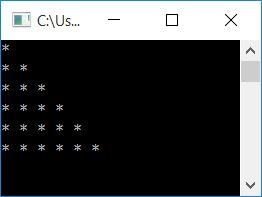The dry run of the for loop of this program goes like this:

• The first statement, or initialization statement, of the for loop gets executed first, but only once. Therefore, 0 gets initialized to i.
• Before entering into the body of the for loop, its condition must be evaluated to be true.
• So the condition i<6 or 0<6 evaluates to be true, therefore program flow goes inside the loop.
• Inside the loop, there is another for loop, so 0 gets initialized to j, and the condition j<=i or 0<=0 evaluates to be true. Therefore, program flow goes inside the loop and prints a * and a space.
• Now the value of j gets incremented. That is, program flow goes to the update part (third statement) of the inner for loop and increments the value of j. So j=1
• Because the condition j<=i or 1<=0 evaluates to false, the execution of this loop is terminated for the time being.
• Now, using endl, a new line is printed, and the next thing begins on the new line.
• The program flow goes to the update part of the outer for loop and increments the value of i. So i=1
• And the condition i<6 or 1<6 again evaluates to be true, therefore program flow goes inside the loop.
• This process continues until its condition (the condition of the outer for loop) evaluates to be false.
• In this way, a half-pyramid pattern using a star (*) gets printed.

To print patterns in C++ programming, you have to use two for loops, the outer for loop and the inner  for loop. The outer for loop is responsible for rows, and the inner for loop is responsible for columns.

## Print an inverted half-pyramid pattern using stars (*)

This C++ program prints an inverted half-pyramid pattern using stars (*).

```#include<iostream>
using namespace std;
int main()
{
int i, j;
for(i=0; i<6; i++)
{
for(j=i; j<6; j++)
cout<<"* ";
cout<<endl;
}
cout<<endl;
return 0;
}```

This program produces another pattern of stars that looks like an inverted half-pyramid of stars, as shown in the sample output given below:## Print the full pyramid pattern of stars (*)

The following C++ program will print a full pyramid pattern of stars (*).

```#include<iostream>
using namespace std;
int main()
{
int i, space, k=0;
for(i=1; i<=6; i++)
{
for(space=1; space<=(6-i); space++)
cout<<"  ";
while(k!=(2*i-1))
{
cout<<"* ";
k++;
}
k=0;
cout<<endl;
}
cout<<endl;
return 0;
}```

This program produces a full pyramid pattern of stars, as shown in the sample output given below:You can also print the full pyramid pattern of stars using the following code instead of the previous one:

```#include<iostream>
using namespace std;
int main()
{
int i, space, j;
for(i=1; i<=6; i++)
{
for(space=6; space>i; space--)
cout<<" ";
for(j=0; j<i; j++)
cout<<"* ";
cout<<endl;
}
cout<<endl;
return 0;
}```

With this C++ code, the pyramid looks like this: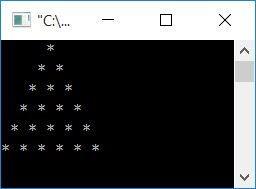## Print an inverted full pyramid pattern using stars (*)

This C++ program prints an inverted full pyramid pattern using stars (*):

```#include<iostream>
using namespace std;
int main()
{
int i, space, j;
for(i=1; i<=6; i++)
{
for(space=1; space<i; space++)
cout<<" ";
for(j=i; j<=6; j++)
{
cout<<"* ";
}
cout<<endl;
}
cout<<endl;
return 0;
}```

This program produces the pattern of stars that looks like an inverted full pyramid, as shown in the snapshot given below:## Print pattern of stars (*) in C++

Based on the previous four programs, we've created a menu-driven program that receives an input from the user as a choice: what the user wants to print. That is, what star pattern does the user want to print:

```#include<iostream>
using namespace std;
void halfPyramid();
void invertedHalfPyramid();
void fullPyramid();
void invertedFullPyramid();
int main()
{
int ch;
do
{
cout<<"1. Print Half Pyramid of Stars\n";
cout<<"2. Print Inverted Half Pyramid of Stars\n";
cout<<"3. Print Full Pyramid of Stars\n";
cout<<"4. Print Inverted Full Pyramid of Stars\n";
cout<<"5. Exit\n";
cout<<"Enter the Choice: ";
cin>>ch;
switch(ch)
{
case 1:
halfPyramid();
break;
case 2:
invertedHalfPyramid();
break;
case 3:
fullPyramid();
break;
case 4:
invertedFullPyramid();
break;
case 5:
return 0;
default:
cout<<"\nWrong Choice!";
break;
}
}while(ch>=1 && ch<=4);
cout<<endl;
return 0;
}
void halfPyramid()
{
int i, j;
for(i=0; i<6; i++)
{
for(j=0; j<=i; j++)
cout<<"* ";
cout<<endl;
}
cout<<endl;
}
void invertedHalfPyramid()
{
int i, j;
for(i=0; i<6; i++)
{
for(j=i; j<6; j++)
cout<<"* ";
cout<<endl;
}
cout<<endl;
}
void fullPyramid()
{
int i, space, j;
for(i=1; i<=6; i++)
{
for(space=1; space<=(6-i); space++)
cout<<" ";
for(j=1; j<=i; j++)
cout<<"* ";
cout<<endl;
}
cout<<endl;
}
void invertedFullPyramid()
{
int i, space, j;
for(i=1; i<=6; i++)
{
for(space=1; space<i; space++)
cout<<" ";
for(j=i; j<=6; j++)
cout<<"* ";
cout<<endl;
}
cout<<endl;
}```

Here is its initial output:Now supply the input, say 1, as your choice; if you want to print half a pyramid of stars, here is the sample output after supplying 1 as input and pressing the ENTER key: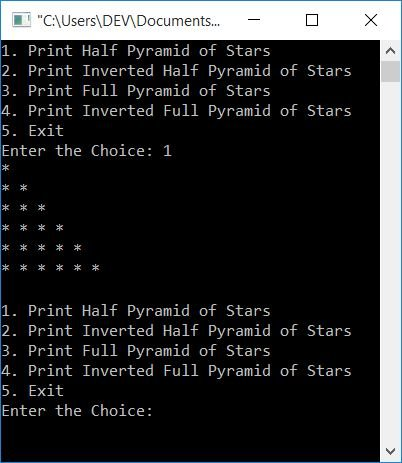As you can see, the half pyramid gets printed, and the menu is again displayed. Now you can operate further; otherwise, if you want to skip or exit, then type 5 or enter 5 and exit from the program of printing a pattern of stars in four ways. Here is another snapshot of the sample run. This snapshot shows the use of all the choices: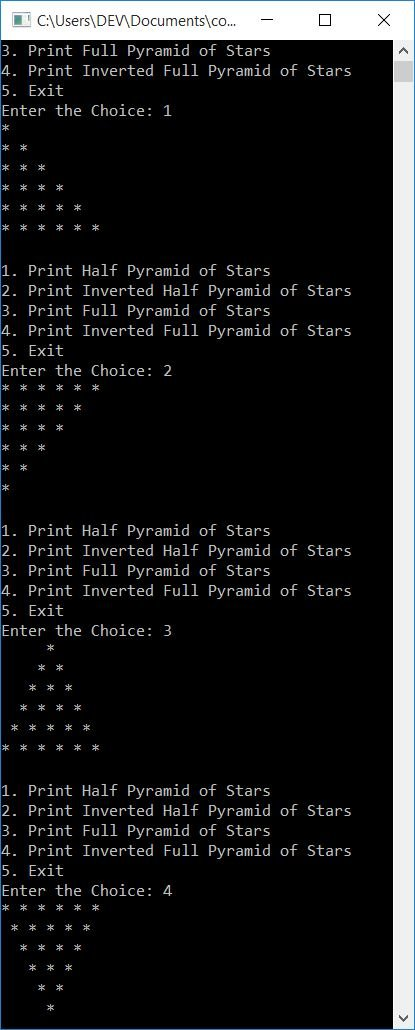This program prints the star pattern (*) with one star in the first row, three stars in the second row, five stars in the third row, and so on up to the sixth row.

```#include<iostream>
using namespace std;
int main()
{
int i, j, k=1;
for(i=0; i<6; i++)
{
for(j=0; j<k; j++)
cout<<"* ";
k=k+2;
cout<<endl;
}
cout<<endl;
return 0;
}```

When the above C++ program is compile and executed, it will produce the following output:Here is another program that prints the same as of previous program, but in an inverted way:

```#include<iostream>
using namespace std;
int main()
{
int i, j, space=20, k=1;
for(i=0; i<6; i++)
{
for(j=0; j<space; j++)
cout<<" ";
space = space-4;
for(j=0; j<k; j++)
cout<<"* ";
k = k+2;
cout<<endl;
}
cout<<endl;
return 0;
}```

The snapshot given below shows the sample output of this C++ program:### Triangle Star Pattern in C++

This C++ program prints a star pattern that looks like a triangle or half-pyramid, whatever you say.

```#include<iostream>
using namespace std;
int main()
{
int i, j, space=10;
for(i=0; i<6; i++)
{
for(j=0; j<space; j++)
cout<<" ";
space = space-2;
for(j=0; j<=i; j++)
cout<<"* ";
cout<<endl;
}
cout<<endl;
return 0;
}```

Here is its sample output, which prints a pattern of stars that looks like a triangle or half-pyramid: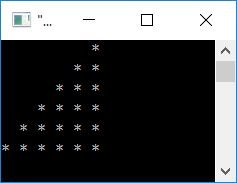### C++ Print a Pyramid Pattern of Stars of a Specific Size

This program receives the input from the user as the size of the pyramid and prints the pattern using stars (*) of that size.

```#include<iostream>
using namespace std;
int main()
{
int i, space, rowSize, k=0;
cout<<"Enter the Number of Rows: ";
cin>>rowSize;
cout<<"\nPyramid of "<<rowSize<<" Rows or Lines:\n";
for(i=1; i<=rowSize; i++)
{
for(space=1; space<=(rowSize-i); space++)
cout<<"  ";
while(k!=(2*i-1))
{
cout<<"* ";
k++;
}
k=0;
cout<<endl;
}
cout<<endl;
return 0;
}```

This is the initial output of the above program's sample run: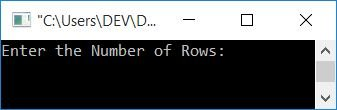Now supply the input, say 6, as the number of rows or lines to print the pyramid pattern that are of 6 lines, as shown in the snapshot given below:Here is another sample run with user input, 10:## Print pattern of numbers in C++

This program prints patterns of numbers (natural numbers). This number pattern looks like a half-pyramid.

```#include<iostream>
using namespace std;
int main()
{
int i, j, num=1;
for(i=0; i<6; i++)
{
for(j=0; j<=i; j++)
{
cout<<num<<" ";
num++;
}
cout<<endl;
}
cout<<endl;
return 0;
}```

This program produces a pattern of numbers that can also be called a half-pyramid of natural numbers, as shown in the following snapshot: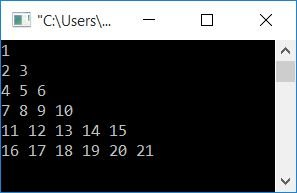Here is another program that does the same job as the previous program. The only difference is that this program begins each row with 1 rather than natural numbers from the first row, first column, to the last row, last column:

```#include<iostream>
using namespace std;
int main()
{
int i, j, num;
for(i=0; i<6; i++)
{
num=1;
for(j=0; j<=i; j++)
{
cout<<num<<" ";
num++;
}
cout<<endl;
}
cout<<endl;
return 0;
}```

The snapshot given below shows the sample output of this program: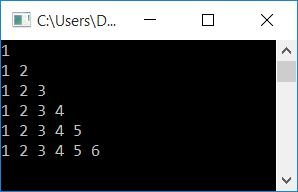## C++ Print Pattern of Alphabets

This C++ program prints patterns of alphabets. That is, a half-pyramid using continuous alphabet characters starting from "A" gets printed using the following program:

```#include<iostream>
using namespace std;
int main()
{
int i, j;
char ch='A';
for(i=0; i<6; i++)
{
for(j=0; j<=i; j++)
{
cout<<ch<<" ";
ch++;
}
cout<<endl;
}
cout<<endl;
return 0;
}```

Here is the sample output of this C++ program to print patterns of alphabets: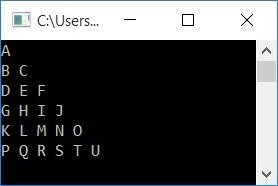Here is another program that starts with a new alphabet character in each row and prints in repeated order for all columns:

```#include<iostream>
using namespace std;
int main()
{
int i, j;
char ch='A';
for(i=0; i<6; i++)
{
for(j=0; j<=i; j++)
{
cout<<ch<<" ";
}
ch++;
cout<<endl;
}
cout<<endl;
return 0;
}```

This program produces the following output: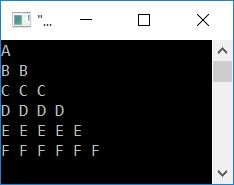#### The same program in different languages

C++ Quiz

« Previous Program Next Program »